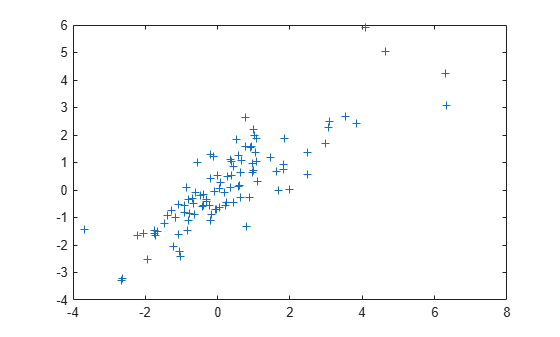# mvtrnd

Multivariate t random numbers

## Syntax

```R = mvtrnd(C,df,cases) R = mvtrnd(C,df) ```

## Description

`R = mvtrnd(C,df,cases)` returns a matrix of random numbers chosen from the multivariate t distribution, where `C` is a correlation matrix. `df` is the degrees of freedom and is either a scalar or is a vector with `cases` elements. If `p` is the number of columns in `C`, then the output `R` has `cases` rows and `p` columns.

Let `t` represent a row of `R`. Then the distribution of `t` is that of a vector having a multivariate normal distribution with mean 0, variance 1, and covariance matrix `C`, divided by an independent chi-square random value having `df` degrees of freedom. The rows of `R` are independent.

`C` must be a square, symmetric and positive definite matrix. If its diagonal elements are not all 1 (that is, if `C` is a covariance matrix rather than a correlation matrix), `mvtrnd` rescales `C` to transform it to a correlation matrix before generating the random numbers.

`R = mvtrnd(C,df)` returns a single random number from the multivariate t distribution.

## Examples

collapse all

Generate random numbers from a multivariate t distribution with correlation parameters `SIGMA = [1 0.8;0.8 1]` and 3 degrees of freedom.

```rng default; % For reproducibility SIGMA = [1 0.8;0.8 1]; R = mvtrnd(SIGMA,3,100);```

Plot the random numbers.

```figure; plot(R(:,1),R(:,2),'+')```